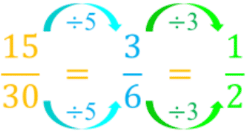# Equivalent fractions#### All in One Place

Everything you need for JC, LC, and college level maths and science classes.#### Learn with Ease

We’ve mastered the national curriculum so that you can revise with confidence.#### Instant Help

24/7 access to the best tips, walkthroughs, and practice exercises available.

0/2
##### Intros
###### Lessons
1. Introduction to Equivalent Fractions
2. What are equivalent fractions?
3. What are fractions in lowest terms (simplest form)?
0/10
##### Examples
###### Lessons
1. Equivalent fractions on number lines
Write the equivalent fractions shown on the number line. Use the equal (=) sign in the answer.
1.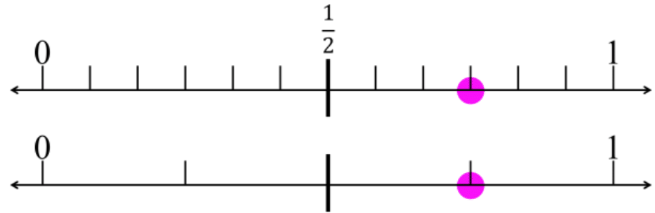2.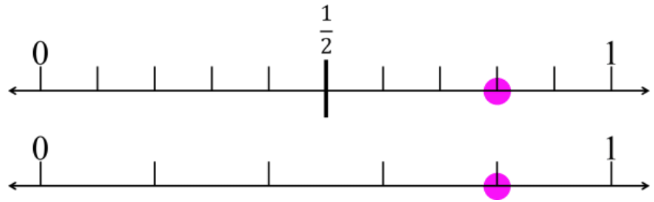2. Equivalent fractions: fill in the blank
Write the missing value to make the fractions equal.
1. $\large \frac{1}{2}$ = $\large \frac{?}{18}$
2. $\large \frac{?}{3}$ = $\large \frac{14}{21}$
3. $\large \frac{1}{4}$ = $\large \frac{25}{?}$
4. $\large \frac{4}{?}$ = $\large \frac{16}{20}$
1. $\large \frac{2}{20}$ = $\large \frac{?}{?}$
2. $\large \frac{70}{140}$ = $\large \frac{?}{?}$
3. $\large \frac{16}{24}$ = $\large \frac{?}{?}$
4. $\large \frac{27}{36}$ = $\large \frac{?}{?}$
0%
##### Practice
###### Free to Join!
StudyPug is a learning help platform covering math and science from grade 4 all the way to second year university. Our video tutorials, unlimited practice problems, and step-by-step explanations provide you or your child with all the help you need to master concepts. On top of that, it's fun - with achievements, customizable avatars, and awards to keep you motivated.
• #### Easily See Your ProgressWe track the progress you've made on a topic so you know what you've done. From the course view you can easily see what topics have what and the progress you've made on them. Fill the rings to completely master that section or mouse over the icon to see more details.
• #### Make Use of Our Learning Aids###### Practice Accuracy

Get quick access to the topic you're currently learning.

See how well your practice sessions are going over time.

Stay on track with our daily recommendations.

• #### Earn Achievements as You LearnMake the most of your time as you use StudyPug to help you achieve your goals. Earn fun little badges the more you watch, practice, and use our service.
• #### Create and Customize Your AvatarPlay with our fun little avatar builder to create and customize your own avatar on StudyPug. Choose your face, eye colour, hair colour and style, and background. Unlock more options the more you use StudyPug.
###### Topic Notes

In this lesson, we will learn:

• How to find equivalent fractions using models
• How to find equivalent fractions using multiplication (and division)
• How to find fractions in lowest terms (simplest form)

Notes:

• Equivalent fractions are fractions with the same value even though they look different (top and bottoms are not the same numbers)
• This can be proven by showing fraction models
• By using the same whole shape and splitting into different numbers of equal parts, all the shape models have the same proportion of shaded area: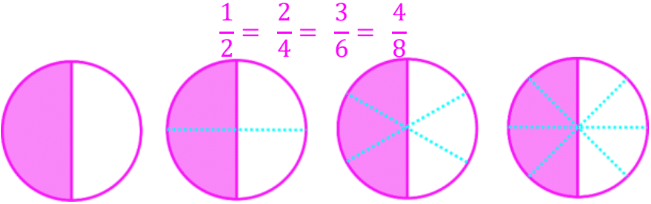• By lining up number lines on top of each other, all the number line models show the dot representing the fractions on the same point along the line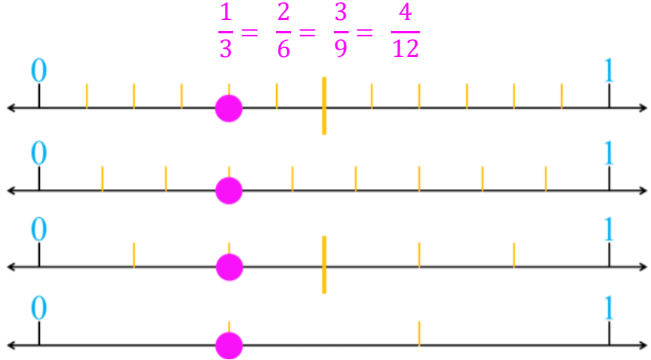• Equivalent fractions have the same value because they take the same fraction (proportion) and multiply BOTH the top and bottom by the same number; the value does not change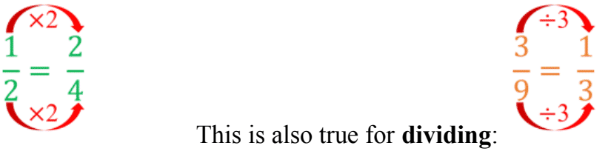• Fractions in lowest terms (or simplest form) are the smallest equivalent fraction
• Divide the top and bottom by the same common factor until you can’t anymore